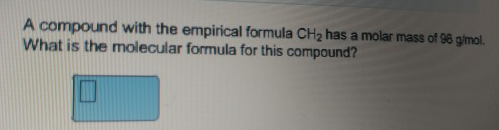# Problem: A compound with the empirical formula CH2 has a molar mass of 98 g/mol. What is the molecular formula for this compound?

###### FREE Expert Solution

The empirical formula of CH2 has a molar mass of 14.03 g/mol. By dividing this to the molecular formula of 98 g/mol. 98/14 = 7

85% (178 ratings)###### Problem Details

A compound with the empirical formula CH2 has a molar mass of 98 g/mol. What is the molecular formula for this compound?